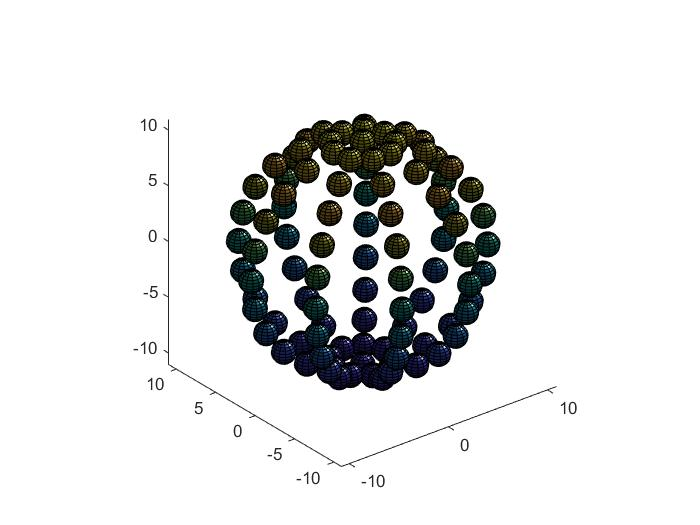# I want better packed sphere

22 views (last 30 days)
ka on 8 Jun 2021
Commented: Rena Berman on 29 Jun 2021 at 14:18
I'm trying to make a spherical shell using smaller spheres, I've reached to this point where these smaller spheres are widely spread, I want to reach at a better packing when no two smaller spheres overlap, can you all help me with this?
here is code for reference,
r = 1;
R = 10;
[x, y, z] = sphere(12);
figure;
Lim = [-R-r, R+r];
axes('NextPlot', 'add', 'XLim', Lim, 'YLim', Lim, 'ZLim', Lim);
axis equal;
view(3);
light;
for alpha = linspace(0, 2*pi,10)
for theta = linspace(0, pi, 13)
xs = sin(theta) * sin(alpha) * R;
ys = sin(theta) * cos(alpha) * R;
zs = cos(theta) * R;
surf(x * r + xs, y * r + ys, z * r + zs);
end
endRena Berman on 29 Jun 2021 at 14:18

Adam Danz on 13 Jun 2021
This file exchange submission offers a method of uniform sampling along the surface of a sphere. After looking at the documentation to understand how to use the functions, all you need to do is specify a density based on the diameter of the smaller spheres. It will return the coordinates of the center points of each small circle.
ka on 14 Jun 2021
I got it.